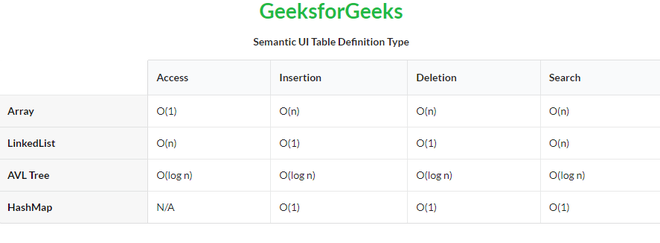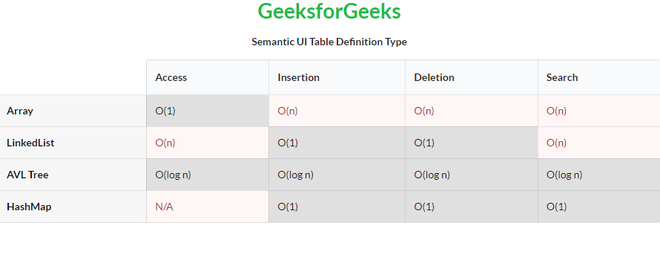GFG App
Open AppBrowser
Continue

# Semantic-UI Table Definition Type

Semantic UI is an open-source framework that uses CSS and jQuery to build great user interfaces. It is the same as a bootstrap for use and has great different elements to use to make your website look more amazing. It uses a class to add CSS to the elements.

Tables are an easy way to organize a lot of data. A table is an arrangement of data in rows and columns, or possibly in a more complex structure. Tables are widely used in communication, research, and data analysis. Tables are useful for various tasks such as presenting text information and numerical data. It can be used to compare two or more items in the tabular form layout. Tables are used to create databases. An HTML table and a Semantic UI table both are the same structurally.

In case of a Definition Type of table, it is formatted to emphasize a first column that defines the content of a row.

Semantic UI Table Definition Type Class:

• definition: This class defines that the type of table is the definition.

Syntax:

```<table class="ui definition table">
<tr>
<td></td>
...
</tr>
...
</table>```

Example 1: This is a basic example illustrating Table Definition Type made using Semantic UI.

## HTML

 ` ` `<``html``> ` ` `  `<``head``> ` `  ``<``title``>Semantic UI Table Definition Type ` ` `  `  ``<``link` `href``= ` `"https://cdnjs.cloudflare.com/ajax/libs/semantic-ui/2.4.1/semantic.min.css"` `rel``=``"stylesheet"``/> ` ` `  `  ``<``script` `src``= ` `"https://code.jquery.com/jquery-3.1.1.min.js"` `integrity``= ` `"sha256-hVVnYaiADRTO2PzUGmuLJr8BLUSjGIZsDYGmIJLv2b8="` `crossorigin``=``"anonymous"``>  ` `  `` ` ` `  `  ``<``script` `src``= ` `"https://cdnjs.cloudflare.com/ajax/libs/semantic-ui/2.4.1/semantic.min.js"``> ` `  `` ` ` ` ` `  `<``body``> ` `  ``<``center``> ` `    ``<``h1` `class``=``"ui green header"``>GeeksforGeeks ` `    ``<``strong``>Semantic UI Table Definition Type ` `  `` ` ` `  `  ``<``table` `class``=``"ui definition table celled"``> ` `    ``<``thead``> ` `      ``<``tr``><``th``> ` `      ``<``th``>Access ` `      ``<``th``>Insertion ` `      ``<``th``>Deletion ` `      ``<``th``>Search ` `    `` ` `    ``<``tbody``> ` `      ``<``tr``> ` `      ``<``td``>Array ` `      ``<``td``>O(1) ` `      ``<``td``>O(n) ` `      ``<``td``>O(n) ` `      ``<``td``>O(n) ` `      `` ` `      ``<``tr``> ` `      ``<``td``>LinkedList ` `      ``<``td``>O(n) ` `      ``<``td``>O(1) ` `      ``<``td``>O(1) ` `      ``<``td``>O(n) ` `      `` ` `      ``<``tr``> ` `      ``<``td``>AVL Tree ` `      ``<``td``>O(log n) ` `      ``<``td``>O(log n) ` `      ``<``td``>O(log n) ` `      ``<``td``>O(log n) ` `      `` ` `      ``<``tr``> ` `      ``<``td``>HashMap ` `      ``<``td``>N/A ` `      ``<``td``>O(1) ` `      ``<``td``>O(1) ` `      ``<``td``>O(1) ` `      `` ` `    `` ` `  `` ` ` ` ` `  ``

Output:Example 2: This is a basic example illustrating Table Definition Type with different States made using Semantic UI.

## HTML

 ` ` `<``html``> ` ` `  `<``head``> ` `    ``<``title``>Semantic UI Table Definition Type ` `     `  `    ``<``link` `href``= ` `"https://cdnjs.cloudflare.com/ajax/libs/semantic-ui/2.4.1/semantic.min.css"`  `    ``rel``=``"stylesheet"``/> ` ` `  `    ``<``script` `src``= ` `"https://code.jquery.com/jquery-3.1.1.min.js"` `integrity``= ` `"sha256-hVVnYaiADRTO2PzUGmuLJr8BLUSjGIZsDYGmIJLv2b8="` `crossorigin``=``"anonymous"``> ` `    `` ` ` `  `    ``<``script` `src``= ` `"https://cdnjs.cloudflare.com/ajax/libs/semantic-ui/2.4.1/semantic.min.js"``> ` `    `` ` ` ` ` `  `<``body``> ` `    ``<``center``> ` `        ``<``h1` `class``=``"ui green header"``>GeeksforGeeks ` `        ``<``strong``>Semantic UI Table Definition Type ` `    `` ` `    ``<``table` `class``=``"ui definition table celled"``> ` `        ``<``thead``> ` `            ``<``tr``><``th``> ` `            ``<``th``>Access ` `            ``<``th``>Insertion ` `            ``<``th``>Deletion ` `            ``<``th``>Search ` `        `` ` `        ``<``tbody``> ` `            ``<``tr``> ` `            ``<``td``>Array ` `            ``<``td` `class``=``"active"``>O(1) ` `            ``<``td` `class``=``"error"``>O(n) ` `            ``<``td` `class``=``"error"``>O(n) ` `            ``<``td` `class``=``"error"``>O(n) ` `            `` ` `            ``<``tr``> ` `            ``<``td``>LinkedList ` `            ``<``td` `class``=``"error"``>O(n) ` `            ``<``td` `class``=``"active"``>O(1) ` `            ``<``td` `class``=``"active"``>O(1) ` `            ``<``td` `class``=``"error"``>O(n) ` `            `` ` `            ``<``tr``> ` `            ``<``td``>AVL Tree ` `            ``<``td` `class``=``"active"``>O(log n) ` `            ``<``td` `class``=``"active"``>O(log n) ` `            ``<``td` `class``=``"active"``>O(log n) ` `            ``<``td` `class``=``"active"``>O(log n) ` `            `` ` `            ``<``tr``> ` `            ``<``td``>HashMap ` `            ``<``td` `class``=``"error"``>N/A ` `            ``<``td` `class``=``"active"``>O(1) ` `            ``<``td` `class``=``"active"``>O(1) ` `            ``<``td` `class``=``"active"``>O(1) ` `            `` ` `        `` ` `    `` ` ` ` ` `  ``

Output:My Personal Notes arrow_drop_up